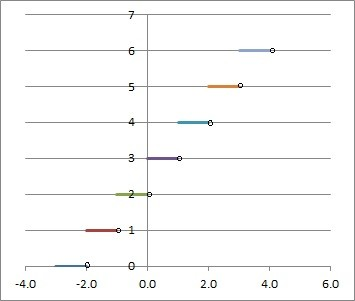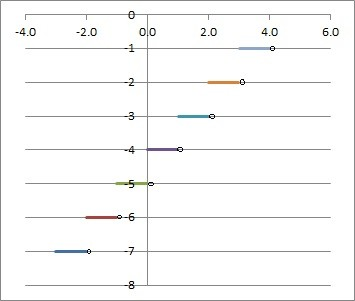# What Is The Floor Function? (3 Key Things To Remember)

The floor function takes a set of real numbers and returns whole numbers, without any fractions or decimals.  However, it is a good idea to know exactly how it works.

So, what is the floor function?  The floor function floor(x) (or x) takes a real number x as input and returns an integer y as output. The output y is the greatest integer less than or equal to x. That is, y = floor(x) is the integer where y <= x and y + 1 > x. For example, floor(1.3) = 1, floor(1.7) = 1, and floor(2) = 2.

Of course, for nonnegative values of x (x >= 0), taking the floor of x is the same as truncating x (cutting off the decimal part).

In this article, we will talk about what the floor function is, what it looks like on a graph, and some of the variations of the graph.  We’ll also answer some common questions about the floor function.

Let’s get started.

## What Is The Floor Function?

The floor function floor(x) is also called the greatest integer function.  It is sometimes denoted by the floor function symbol ⌊x⌋.

The floor function y = floor(x) takes a real number x as input (so the domain is the set of all real numbers).

The output y of the floor function is an integer y.  The output y is the greatest integer less than or equal to x.

In other words, y is the unique integer that satisfies the equation y <= x < y + 1.

The floor function behaves a little differently depending on whether x is negative or not:

• For nonnegative values of x (x >= 0), floor(x) is the same as truncating x (removing the decimal or fraction part).
• For negative values of x, floor(x) + 1 = trunc(x).  Alternatively, ceiling(x) = trunc(x) for x < 0.

### What Does The Floor Function Do?

The floor function takes a real number (which may contain decimals or fractions) as input and returns an integer (whole number) without decimals or fractions as the output.

In other words, floor(x) finds the closest integer to x that is less than or equal to x.  For a given real number x, there are infinitely many integers less than or equal to x, but floor(x) is the largest one of those.

### How Do You Find The Floor Of A Number?

Here are the steps to find the floor of a number x:

• If x is an integer (a whole number with no fractions or decimals), then floor(x) = x.
• If x is not an integer, look at the sign of x (positive or negative).
• If x is positive, then truncate x (cut off the decimal or fraction part) to get floor(x).
• If x is negative, then truncate x and subtract 1 to get floor (x).

#### Example 1: Floor Of 6

Since 6 is an integer, floor(6) = 6.

#### Example 2: Floor Of 4.5

Since 4.5 is not an integer, we look at the sign, which is positive.

Then we truncate 4.5 to 4.

So floor(4.5) = 4.

#### Example 3: Floor Of -2.5

Since -2.5 is not an integer, we look at the sign, which is negative.

Then we truncate -2.5 to -2.

Then we subtract 1 to get -3.

So floor(-2.5) = -3.

### Floor Function Formula

We can write a formula for the floor function in a couple of different ways.

One way to write floor(x) is by using the truncate function.  There is one part for x >= 0 and one part for x < 0:

• floor(x) = {truncate(x), x >= 0 or x a negative integer; truncate(x) – 1, x < 0 and x not an integer}

Another way to write floor(x) is by using integers:

• For each integer n, floor(x) = n for n <= x < n + 1.

### Floor Function Graph

Here is the graph of the floor function, y = floor(x) or y= x

Note that the graph looks somewhat like a staircase.

Also, note that the graph has a jump discontinuity at every integer value of x (x = … -2, -1, 0, 1, 2, …)

Finally, observe that the y-values of the floor function are all integers (no fractions or decimals).

### Floor Function Examples

Here are some examples of floor functions and modifications based scaling and shifts (horizontal and vertical).

#### Example 1: Floor Function With A Scale

Consider the scaled floor function f(x) = 2*floor(x).

This looks like the regular floor function (graphed earlier), but it is twice as tall (every y-value is doubled from the basic floor function).

#### Example 2: Floor Function With A Vertical Shift

Consider the floor function f(x) = floor(x) + 3

This looks like the regular floor function (graphed earlier), but it has vertical shift: it is moved up 3 units from the basic floor function.This is the graph of the floor function with a vertical shift of 3 units up: f(x) = floor(x) + 3.

#### Example 3: Floor Function With A Horizontal Shift

Consider the floor function f(x) = floor(x – 4)

This looks like the regular floor function (graphed earlier), but it has horizontal shift: it is moved right 4 units from the basic floor function.This is the graph of the floor function with a horizontal shift of 4 units to the right: f(x) = floor(x – 4).

#### Example 4: Floor Function With Scaling & Shifts

Consider the floor function f(x) = 2*floor(x – 4) + 3

This looks like the regular floor function (graphed earlier), but it has 3 aspects that change it:

• A horizontal shift of 4 units to the right.
• A scaling (doubled), giving it twice the height.
• A vertical shift of 3 units up.

### Is The Floor Function Injective (One-To-One)?

The floor function is not injective, since there are always multiple x-values (inputs) that lead to the same output (y-value).

For example, for a given output value of y (an integer), both of the inputs y + 0.1 and y + 0.2 have a floor of y, which means that floor(y + 0.1) = y and floor(y + 0.2) = y.

Thus, floor(x) is not an injective function.

### Is The Floor Function Surjective (Onto)?

The floor function floor(x) is not surjective onto the set of real numbers.  Remember that the outputs of the basic floor function are only integers (whole numbers with no fractions or decimals).

The floor function is surjective onto the set of integers.

### Is The Floor Function Invertible?

The floor function is not invertible, since it is not one-to-one or onto.

### Is The Floor Function Continuous?

The floor function is not continuous, since it has a discontinuity at every integer value of x.

However, floor(x) is continuous on every interval between integers.  That is, floor(x) is continuous on the interval (n, n+1) for each integer n.

### Is The Floor Function Differentiable?

The floor function is not differentiable, since it has a discontinuity at every integer value of x (and a function must be continuous to be differentiable).

However, floor(x) is differentiable on every interval between integers.  The derivative of floor(x) is 0 on the interval (n, n+1) for each integer n.

### Is The Floor Function Even Or Odd?

The floor function is neither even nor odd.  Here is the proof:

Take x = 1.5.  Then:

• floor(x) = floor(1.5) = 1
• floor(-x) = floor(-1.5) = -2
• -floor(x) = -floor(1.5) = -1

Since floor(-x) is not equal to floor(x), the floor function is not even.

Since floor(-x) is not equal to -floor(x), the floor function is not odd.

### What Is The Floor Function In Excel?

The floor function in Excel is given by FLOOR, and it has 2 inputs:

• The first input is a number or a cell that contains a number.
• The second input is a significance level.  To get an integer output, use 1 for this input.

So, to find the floor of the number x in Excel, use the formula

• =FLOOR(x, 1)

If x in in cell A1, we would use the formula

• =FLOOR(A1, 1)

### Floor vs Truncate

Remember that the definitions are as follows:

• The floor function returns the greatest integer than is less than or equal to x.
• The truncate function cuts off the decimal or fraction part of a number x, leaving only the integer part.

For nonnegative values of x (x >= 0), the floor and truncate functions will return the same value.  In other words, floor(x) = trunc(x) for x >= 0.

For negative values of x (x < 0), there are two cases:

• Case 1: x is an integer: in this case, the floor and truncate functions are the same.
• Case 2: x is not an integer: in this case, the floor and truncate functions are one unit apart (truncate is larger).  In other words, floor(x) = trunc(x) – 1 when x is negative and not an integer.

The following table shows some values of x, along with the outputs for both the floor and truncate functions.

## Conclusion

Now you know what the floor function is, how it works, and what it looks like on a graph.  You also know the answers to some common questions about floor functions.

You can learn about the ceiling function here.